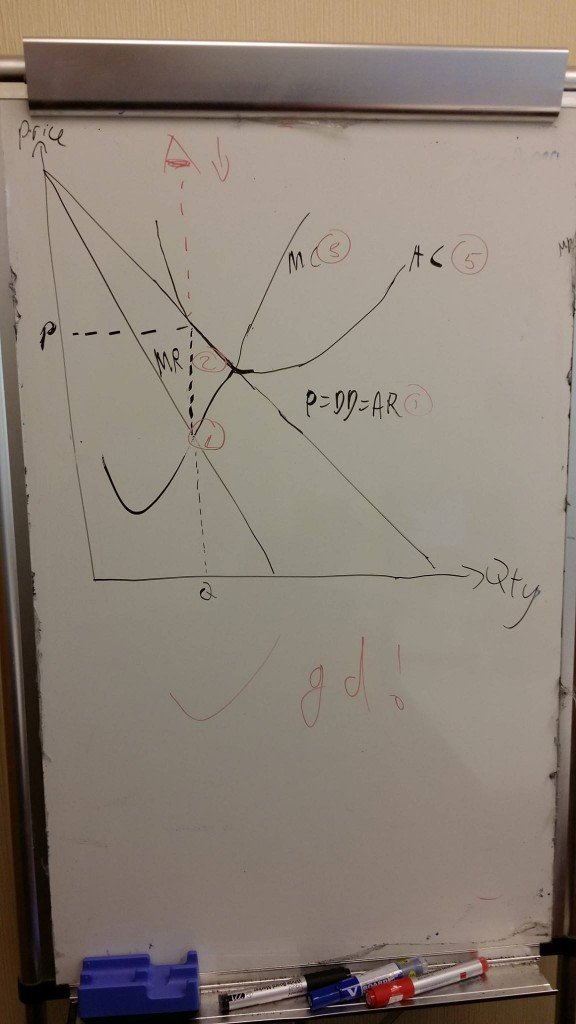# Draw a perfect market structure diagramWant to know the exact 6 step approach to draw a perfect market structure diagram for your A level Econs?

Yes, drawing of a perfect market structure diagram calls for specifically 6 steps, which will shorten your drawing time from your usual 10 mins to 2 mins.

First, you need to identify the above market structure, which is a monopoly or monopolistic competitive firm.

Step 1 – Draw the downward sloping demand curve, which is also equal to the Average Revenue;

Step 2 – Draw the Marginal Revenue curve, which is exactly half the size of the Average Revenue;

Step 3 – Draw the Marginal Cost curve, which is a nike tick;

Step 4 – Identify the golden intersection where Marginal Cost = Marginal Revenue. This is the profit maximizing point and all students MUST know;

Step 5 – Insert your Average Cost curve. The position of AC curve will determine whether the firm is making profit / loss or breaking even. The higher the position of AC curve, the higher the LOSS. One key note is that the Marginal Cost curve will have to intersect the AC curve at the minimum point!

Step 6 – Look vertically upwards where your profit maximizing output is. The area between your AC and AR will be your profit / loss.

Simple enough? If you still can’t figure out, feel free to join my classes and I will explain in depth to you!